Feel free to send e-mail to me anytime. I'm always ready to hear your comments, answer questions about class or help you with homework.

ANNOUNCEMENTS

# Click here to see how the class did.

 This page is now complete. (The class results and grade distribution will be posted after the quarter, however.) Sample Final solution posted. Extra problems and solutions posted. See Samples page Review Session: Sunday, December 7, 2:30 - 4:30 PM in in 1153 Smith Laboratory. Finals Week Office hours: Monday and Tuesday, 1:30 - 3:00.

• Nov 28. Answer clue to 40-Q4 has been corrected.
• Nov 4. Review session @ 6:00 - 7:48 PM in 100 Independence Hall.
Oct 23.
The answer clue for problem 35-28 was incorrect. It has been fixed.
• Oct 8. Important changes for next week:
*** Extra office hours tomorrow, Thursday, from 12:00 to 1:30 to help get ready for the Midterm.
*** No office hours Monday.
*** The review session has changed to Wednesday, October 15, 6:30 to 8:18 pm in Room 1153 Smith Lab (the lecture room).
• Sept 24. Office hours have changed from what was given in the General Information Sheet: They are: MW 1:30 to 3:00.
• Sept 24. Here are the handouts provided in class today if you didn't get one: syllabus and sines and cosines.
• Sept 24. First day of class.

JUMP STATION

 Homework Assignments and Solutions Answer Clues Anonymous Comments Check your scores on WebCT

 Class info: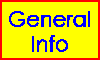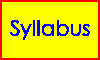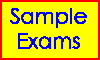Computer aids: VIRTUAL DEMOS ActivPhysics: Waves ActivPhysics: Light ActivPhysics: Relativity and Quantum Mechanics Class related: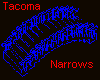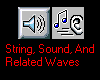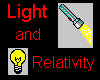Other Links: Treasure Trove Of Physics The Math Zone History of the Universe Windows to the Universe AIP Physics News (searchable) My Home Page OSU Physics Home Page OSU Home Page

SOLUTIONS

 This section provides solutions to exam, selected recitation, and homework problems. The solutions are stored as Portable Document Format (PDF) files. Your browser may already incorporate a PDF viewer, but if not, you can get one called Acrobat Reader free from Adobe Systems Incorporated.

 #1 due September 30 #2 due October 7 #3 due October 14 #4 due October 21 #5 due October 28 #6 due November 4 #7 due November 13 #8 due November 18 #9 due November 25 and Twin Paradox pages 5-7 #10 due December 2
 Week #1 September 25 Week #2 September 30 Week #3 October 7 Week #5 October 21 and 23 Week #6 October 28 Week #8 November 13 Week #9 November 18: Twin Paradox pages 1-4. Week #10 November 25 Week #11 December 2

 Quiz 1 Quiz 2 Quiz 3 Quiz 4 Quiz 5

Back to the Jump Station

HW
Answers or clues to most homework problems.
Yellow marks the current assignment.

#1
 Q3 (a) #2 (b) positive Q7 (a) pi (b) pi 3 T = 0.5s, k = 79 N/m, vm = 4.40 m/s, Fm = 27.6 N 12 x = 3 m, v = -49 m/s, a = -270 m/s/s, phase = 20 rad 20 xm = 0.5m, x = -0.251 m, v = 3.06 m/s 22 2.1 rad 33 (b) 125 J (c) 250 J (d) Use one of the special points (an endpoint or equilibrium) of SHM. 36 xm = mv / sqrt[k(M+m)] S84 9.47 m/s/s S86 Solve for k in terms of m,g,h using Newton's 2nd Law. 55 froom = 0.35 Hz, felevator,up = 0.39 Hz, felevator,down = 0
#2
 Q5(a) wave #4 2(a) 400 nm -> 7.5 x 10^14 Hz 700 nm -> 4.3 x 10^14 Hz S57 v = 200 cm/s S64 vt = 94 mm/s 8 v = 2.0 cm/s; the phase constant in the wave equation is pi, not zero; vt = -2.5 cm/s 16 vm = 300 m/s S60 (a) P2 = 2*P1 (b) P2 = P1/4 26 phi = 1.45 rad 0.23 lambda 33 v = 144 m/s lambda = 60 cm f = 241 Hz 38 There are two harmonics that work. One is the 4th harmonic.

#3
 Q14(a) V3 > V2 = V1 3 the listener 5 1900 km 10 v = 6.0 m/s 13 fmin,1 = 343 Hz and fmax,1 = 686 Hz. In the allowed range, there are 28 frequencies for destructive interference (fmin,1 to fmin,28) 16 r = 0.175 m 22 39.5 dB 28 The intensity at the microphone is 5.97x10^(-5) W/m^2 S75a v = 170 m/s S76 (a) 77.6 Hz (b) 77.0 Hz

#4
 Q7 na > nb 2 (a) 0.50 ms (b) 503 s S82 B points in the positive x direction when E points in the positive y direction (note that the light is moving in the negative z direction). Don't forget that all of our basic wave equation results apply for light. We just need to use v = c = 3.00 x 10^(8) m/s. 9 B = -y Bo cos (same phase factor as E) Bo = 6.7 x 10^(-9) T, f = 0.5 x 10^(15) Hz 45 For the observer to see the corner, a ray from the corner must refract sideways when it hits the upper-left corner. n = 1.26. S69 diameter = 4.56 m 53 nglass >= 1.22 57 You must block the rays that are not totally reflected. 63% gets covered.

#5
 Q1 Decide first how the index of refraction should vary with height. To do this, divide the air into horizontal layers and analyze what happens between layers. Then use the fact that nair increases if the temperature decreases. 1 40 cm 6 1.5 m 18 1.86 mm 27 The first lens has an image that is real and inverted. This image becomes the object for the second lens, and it is a virtual object because it is on the "wrong" side. The final image is virtual and has the same orientataion as the object. S60 Start by finding the image of the lens and ignoring the mirror. That image becomes the object for the mirror. The mirror's image becomes the new object for the lens, and it produces the final image. The lens is used twice in this problem. The final image is 0.6 m to the left of the lens, real, and not inverted. 27 The final image is in the same place as the object, virtual, and inverted. 35 For far objects, the retina must be at the focus. For objects that are closer, it won't be. (a) 2.35 cm (b) radius of curvature decreases.

#6
 Q7a,b decrease, decrease 1 f = 5.09 x 10^(14) Hz 5 22.0 degrees 7 The wavelength in a medium that has index of refraction n, is lambda/n. 10 The smallest is 1550 nm. 12 The phase difference for dark fringe m is (2m+1) pi. 13 2.25 mm 20 Determine the path length difference as a function of x. Answer is: 8.75 times lambda 21 "m" tells you the phase difference for a spot, however the difference occurred. Answer = 6640 nm. 27 The furthest is at x = 7.50 m.

#7
 Q6 Assume the light diffracts through some structure in your eye before reaching the retina. (a) larger Q7 The larger a is, the smaller the diffraction central maximum. (a) less 2 0.00750 rad 0.118 mm 4 a = 2.51 mm (that's big!) 6 38 m 10a 0.003143 rad 9 Recall, I is proportional to E^2. First, break the slit into N sections (Huygen wavelets). Each section contributes an electric field wave in phase at the central max. Now, if you double the slit size you'll have 2N sections. 27,28 The spacing between 2-slit interference fringes is constant to good approximation. So is the spacing between diffraction minima. Answer to 28: 3. 31 Use your result from problem 28. 32 d = 20.1 x 10^(-6) m a = 5.04 x 10^(-6) m

#8

 Q4a Sam Q10 (a) 3 > 2 = 1;   (b) 2 > 1 = 3 S58 v = 0.75 c 3 v = 0.99c 6 v = 0.9999995 c 11 87.4 m, 0.394 us 25 1.2 us 26 (a) 1.25 y;   (b) 1.6 y;  (c) 4.0 y 37 18 suns/year S61 Use energy methods for both parts. (a) 256 kV;   (b) 0.746 c

 Q14  Use your answers to Q12. Q16  Use your answers to Q15. Q17  t = toutbound + tinbound, so make sure to break the problem into two parts. Q18  Use the definition of speed. Since you already know the answer from Q7, the point here is to show your work.

#9

 5 Power is energy per unit time. You need to convert to photons per unit time. 8 8.6 x 10^5 m/s 38 At what angle is the shift maximized? 2.65 x 10^(-15) m 43 Back scattering means angle = 180 degrees. (a) Find the change in wavelength first. ans = 41.8 keV 50 1.7 x 10^(-35) m 64 (a) 15 keV 74 Note we are given py = pz = 0, so they are given exactly. 75 0.19 m

#10

 Q2 Case (a) has the largest energy. Q4 This is a standing wave problem, right? (a) Points of zero probability are places where the wave function is always zero. ans = 18 4 0.85 nm 6 0.65 eV 11 An electron in a well can only have certain allowed energies. If it absorbs a photon, the photon's energy must be exactly that necessary so that the electron ends up at one of these allowed energies. This tells you what the allowed energies of the photon are. Just convert photon energy to wavelength to complete the problem. 14 Look at Fig. 40-6. (a) big decrease. (b) small increase. (If you decided that the answer is "no change", that's pretty close.) 17 Use the hint provided in the text. (I'll work part of this in class.)

Back to the Jump Station

You can send anonymous comments to me here.
I will not be able to respond directly back to you, of course, because this is anonymous!

Type your comments in the space below and then hit Send Comments.
Your identity will not be recorded. If you doubt this, use a public machine where you don't need to log in.

You can send e-mail to me here.

Back to the Jump Station